# pH is a logarithmic scale used to indicate the hydrogen ion concentration, [H+], of a solution:...

pH is a logarithmic scale used to indicate the hydrogen ion concentration, [H+], of a solution:

pH=−log[H+]

Due to the autoionization of water, in any aqueous solution, the hydrogen ion concentration and the hydroxide ion concentration, [OH−], are related to each other by the Kw of water:

Kw=[H+][OH−]=1.00×10−14

where 1.00×10−14 is the value at approximately 297 K. Based on this relation, the pH and pOH are also related to each other as

14.00=pH+pOH

Part B

Part complete

0.25 g of sodium hydroxide (NaOH) pellets are dissolved in water to make 7.0 L of solution. What is the pH of this solution?

Express the pH numerically to two decimal places.

Part C

What is the pOH of the solution in Part B?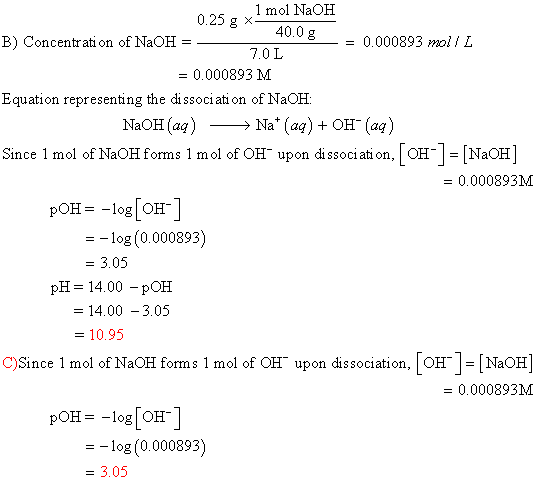##### Add Answer of: pH is a logarithmic scale used to indicate the hydrogen ion concentration, [H+], of a solution:...
Similar Homework Help Questions
• ### NA Review | Constants 1 Periodic Table pH is a logarithmic scale used to indicate the...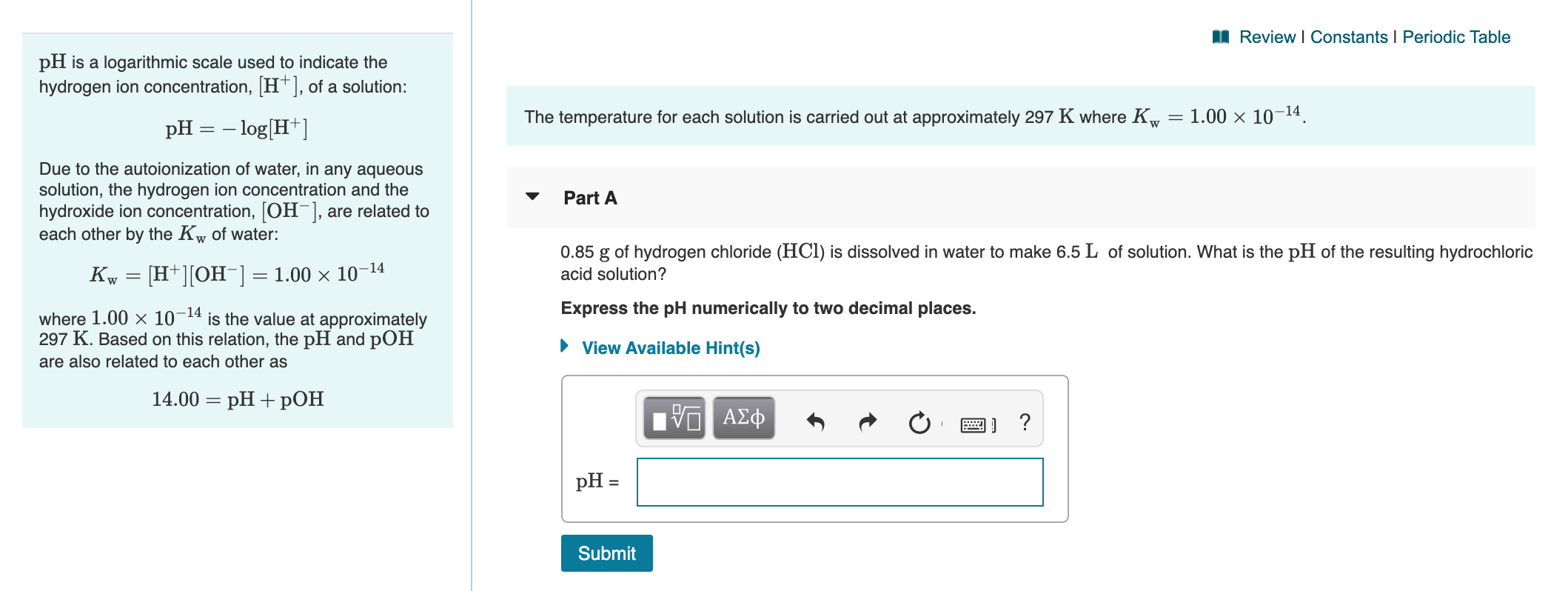NA Review | Constants 1 Periodic Table pH is a logarithmic scale used to indicate the hydrogen ion concentration, [H+], of a solution: The temperature for each solution is carried out at approximately 297 K where Kw = 1.00 x 10-14. pH = -log[H+] Due to the autoionization of water, in any aqueous solution, the hydrogen ion concentration and the hydroxide ion concentration, [OH-], are related to each other by the Kw of water: Part A Kw = [H+][OH-] =...

• ### ± pH of a Strong Acid and a Strong Base pH is a logarithmic scale used...

± pH of a Strong Acid and a Strong Base pH is a logarithmic scale used to indicate the hydrogen ion concentration, [H+], of a solution: pH=−log[H+] Due to the autoionization of water, in any aqueous solution, the hydrogen ion concentration and the hydroxide ion concentration, [OH−], are related to each other by the Kw of water: Kw=[H+][OH−]=1.00×10−14 where 1.00×10−14 is the value at approximately 297 K. Based on this relation, the pH and pOHare also related to each other...

• ### I need answer for C, thanks. Review | Constants 1 Periodic Table pH is a logarithmic...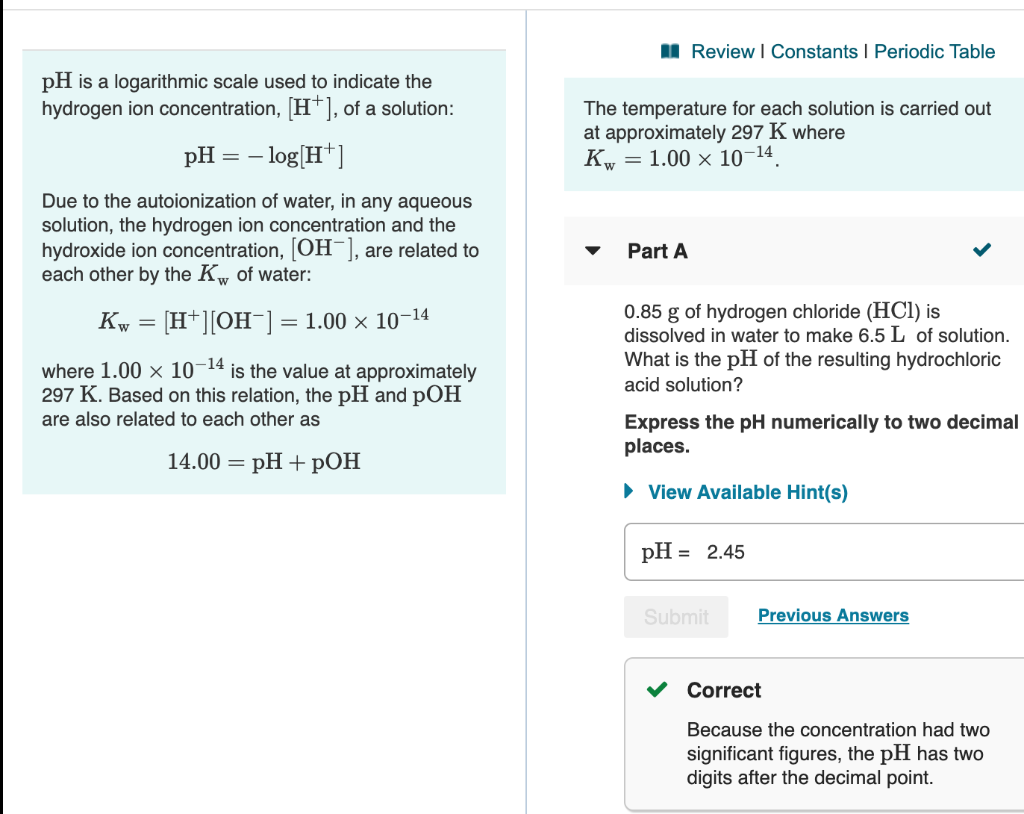I need answer for C, thanks. Review | Constants 1 Periodic Table pH is a logarithmic scale used to indicate the hydrogen ion concentration, (H+), of a solution: The temperature for each solution is carried out at approximately 297 K where Kw = 1.00 x 10-14, pH = -log[H+] Due to the autoionization of water, in any aqueous solution, the hydrogen ion concentration and the hydroxide ion concentration, OH 1, are related to each other by the Kw of water:...

• ### 0.50 g of hydrogen chloride HCl is dissolved in water to make 8.0 L of solution. What is the pH...

is a logarithmic scale used to indicate the hydrogen ionconcentration, , of a solution:Due to the autoionization of water, in any aqueous solution, the hydrogen ion concentration and the hydroxide ion concentration, , are related to each other by the of water:Based on this relation, the and are also related to each other asa)0.50 of hydrogen chloride() is dissolved in water to make 8.0 of solution. What is the of the resulting hydrochloric acid solution?Express the pH numerically.b).25 of sodium...is a logarithmic scale used to indicate the hydrogenionconcentration, , of a solution:Due to the autoionization of water, in any aqueous solution, thehydrogen ion concentration and the hydroxide ion concentration,, are related to each other by the of water:Based on this relation, the and are also related to each other as Part A0.50 of hydrogen chloride () is dissolved in water to make 8.0 of solution. What is the of the resulting hydrochloric acid solution?Express the pH numerically.=Part B0.70 of...

• ### 0.15g of hydrogen chloride (HCL) story Bookmarks People Window Help ® D Thu * C Sign...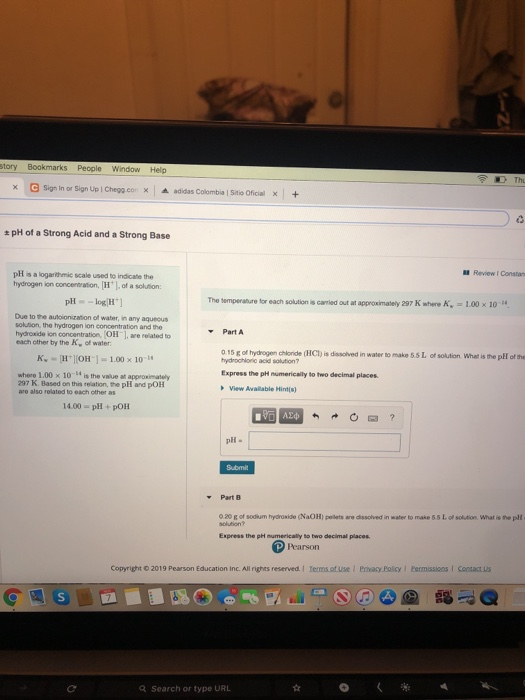0.15g of hydrogen chloride (HCL) story Bookmarks People Window Help ® D Thu * C Sign in or Sign Up | Chegg.con x adidas Colombia Sitio Oficial pH of a Strong Acid and a Strong Base Review Consta pH is a logarithmic scale used to indicate the hydrogen ion concentration, H of a solution: The temperature for each solution is carried out at approximately 297 K where -1.00 x 10-4 pH -log H) Due to the autolocation of water, in...x.øi5le="" alt="rm pH" src="http://session.masteringchemistry.com/render?tex=%5Crm+pH" align="center" /> is a logarithmic scale used to indicate the hydrogen ionconcentration, , of a solution:Due to the autoionization of water, in any aqueous solution, the hydrogen ion concentration and the hydroxide ion concentration, , are related to each other by the of water:Based on this relation, the and are also related to each other asPart A0.50 of hydrogen chloride () is dissolved in water to make 8.0 of solution. What is the of the resulting...

• ### The hydroxide ion concentration in an aqueous solution at 25°C is 4.4x10-2 M. The hydronium ion...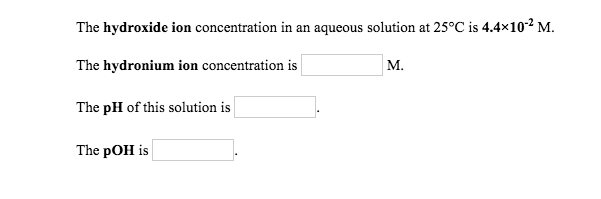The hydroxide ion concentration in an aqueous solution at 25°C is 4.4x10-2 M. The hydronium ion concentration is M. The pH of this solution is The pOH is The hydronium ion concentration in an aqueous solution at 25°C is 4.4x10 M. The hydroxide ion concentration is M. The pH of this solution is The pOH is Autoionization occurs when two solvent molecules collide and a proton is transferred between them. Write the autoionization reaction for water. Submit Answer Use pH,...

• ### A. What is the pH of an aqueous solution with a hydrogen ion concentration of H...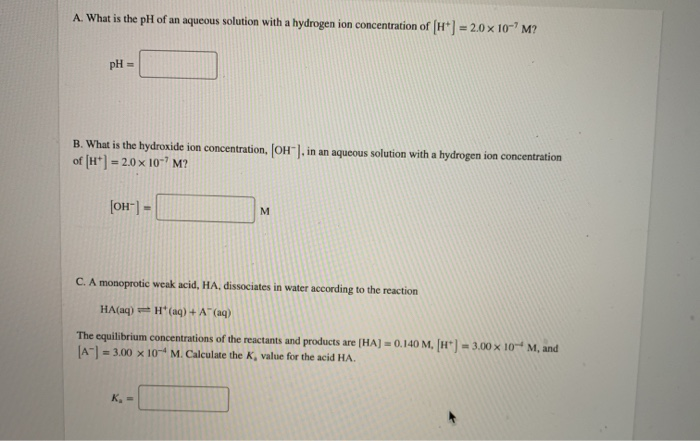A. What is the pH of an aqueous solution with a hydrogen ion concentration of H 20 x 10-'M? pH = B. What is the hydroxide ion concentration, OH"), in an aqueous solution with a hydrogen ion concentration of (H) = 2.0 x 10-'M? [OH-] = C. A monoprotic weak acid, HA, dissociates in water according to the reaction HA(aq) H(aq) + A+ (aq) 3.00 x 10-M, and The equilibrium concentrations of the reactants and products are (HA) = 0.140...

• ### The temperature for each solution is carried out at approximately 297 K where Kw=1.00×10−14. Part...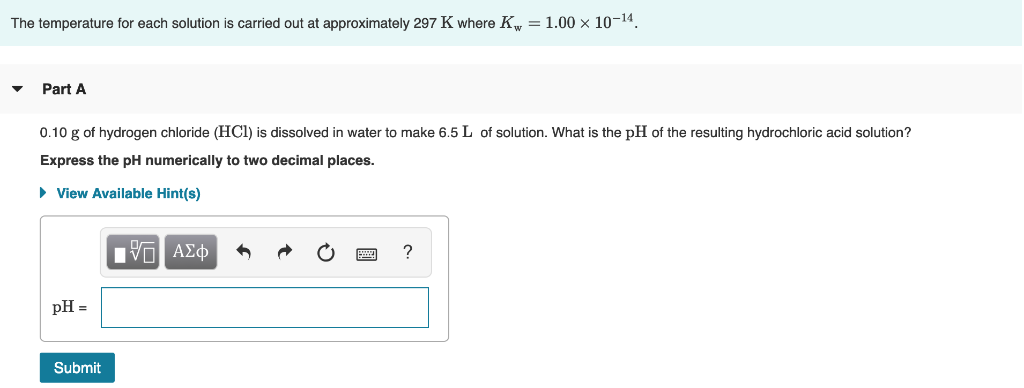The temperature for each solution is carried out at approximately 297 K where Kw=1.00×10−14. Part A 0.10 g of hydrogen chloride (HCl) is dissolved in water to make 6.5 L  of solution. What is the pH of the resulting hydrochloric acid solution? Express the pH numerically to two decimal places. View Available Hint(s) The temperature for each solution is carried out at approximately 297 K where Kw = 1.00 × 10-14 ▼ Part A 0.10 g of hydrogen chloride (HCI)...

Need Online Homework Help?